﻿ Stochastic DEA with a Perfect Object and Its Application to Analysis of Environmental Efficiency

Stochastic DEA with a Perfect Object and Its Application to Analysis of Environmental Efficiency

Alexander Y. VaninskyOPEN ACCESSPEER-REVIEWED

Stochastic DEA with a Perfect Object and Its Application to Analysis of Environmental Efficiency

Alexander Y. VaninskyMathematics Department, City University of New York/Hostos Community College, New York, USA

Abstract

The paper introduces stochastic DEA with a Perfect Object (SDEA PO). The Perfect Object (PO) is a virtual Decision Making Unit (DMU) that has the smallest inputs and greatest outputs. Including the PO in a collection of actual objects yields an explicit formula of the efficiency index. Given the distributions of DEA inputs and outputs, this formula allows us to derive the probability distribution of the efficiency score, to find its mathematical expectation, and to deliver common (group–related) and partial (object-related) efficiency components. We apply this approach to a prospective analysis of environmental efficiency of the major national and regional economies.

At a glance: Figures

1
Prev Next

• Vaninsky, Alexander Y.. "Stochastic DEA with a Perfect Object and Its Application to Analysis of Environmental Efficiency." American Journal of Applied Mathematics and Statistics 1.4 (2013): 57-63.
• Vaninsky, A. Y. (2013). Stochastic DEA with a Perfect Object and Its Application to Analysis of Environmental Efficiency. American Journal of Applied Mathematics and Statistics, 1(4), 57-63.
• Vaninsky, Alexander Y.. "Stochastic DEA with a Perfect Object and Its Application to Analysis of Environmental Efficiency." American Journal of Applied Mathematics and Statistics 1, no. 4 (2013): 57-63.

 Import into BibTeX Import into EndNote Import into RefMan Import into RefWorks

1. Introduction

Data Envelopment Analysis (DEA) estimates the relative efficiency of a group of objects referred to as Decision-Making Units (DMUs) that use inputs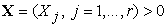to produce outputs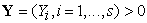. It was developed in publications , , and . DEA combines all of the indicators of each object into an efficiency index E scaled to an interval [0,1]. An object is considered efficient if it receives a score equal to 1, and inefficient if it receives a score of less than 1. The DEA efficiency measure is based on the efficiency ratio suggested in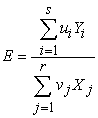(1)

where u = (u1,..., us) ≥ 0 and v = (v1,..., vr) ≥ 0 are the weights assigned to outputs and inputs, respectively. To estimate the weights, DEA sets up a series of optimization problems similar to the following one:

For each DMUk, k = 1,..., N, find vectors uk = (uk1,..., uks) ≥ 0 and vk = (vk1,..., vkr) ≥ 0 such that: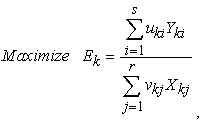(2)

subject to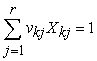, and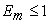for all DMUm, m = 1,…, N, in the group with the same weight coefficients uk = (uk1,…, uks) and vk = (vk1,…, vkr).

Reference  showed that maximization of the efficiency ratio (2) is equivalent to solving a linear programming (LP) problem, one for each DMU in a group:

For each DMUk, k = 1,..., N,

Minimize θ

subject to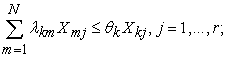(3)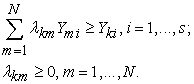The LP algorithm forms a linear combination of DMUs that outperforms the currently selected DMU by both inputs and outputs. Publications [6, 11] provide details and reviews of contemporary DEA.

One of the disadvantages of DEA is that it measures the relative efficiency only. To work around this, some DEA publications suggest a best practice comparison. But all of them remain within the framework of conventional DEA and use the LP algorithm. In publications [17, 18, International Journal of Mathematical Modeling, Simulation and Applications 4(3): 217-233, 2011.">19] explicit formulas for the DEA efficiency scores are obtained, assuming that a Perfect Object (PO) is added to the group of actual DMUs. The PO is a virtual DMU having the smallest inputs and greatest outputs; it serves as a benchmark for efficiency comparisons. The obtained formulas obviate the need to use the LP algorithm and require only a moderate number of simple operations with ratios of inputs and outputs.

Another disadvantage of conventional DEA is its deterministic nature. Some authors claim that the DEA, unable to deal with stochastic noise in inputs and outputs, cannot distinguish between actual inefficiency and short-lived negative effects or plain bad luck. To address these concerns, a stochastic version of DEA (SDEA) was developed in publications [2, 10, 15], to name just a few. SDEA is a rapidly developing dimension of DEA, and new approaches, procedures, and algorithms continue to appear.

Conventional SDEA is aiming to find a region of stochastic dominance or to eliminate the stochastic noise. Since DEA with a Perfect Object (DEA PO) furnishes an explicit formula for the efficiency index, it provides new opportunities for stochastic extension of DEA. Firstly, the explicit formula allows for the computation of the probability density function (pdf) of the efficiency index directly. Secondly, it introduces stochasticity unrelated to imprecision in data measurement. Thirdly, it introduces an alternative breakdown of the efficiency index into two components. The first component is related to the efficiency of a group as a whole, the second - to the relative efficiency of an individual DMU in the group. This decomposition stems from the fact that in the framework of stochastic DEA with a perfect object (SDEA PO), an individual DMU may be considered as an occurrence of a random normalized DMU, having all inputs and outputs scaled to the interval of [0,1]. The mathematical expectation of the normalized DMU’s efficiency, referred to below as common efficiency, is due to fundamental, systemic factors underlying the group. The difference between the observed and common efficiency is called partial efficiency, which is determined by the factors specific to a particular DMU. This paper develops the above-mentioned SDEA PO approach and demonstrates its application.

The paper is organized as follows. In Section 2, stochastic DEA with a perfect object is developed, and Section 3 presents its application to the analysis of prospective environmental performance of the major economies and regions. The appendix provides a computer program written in the R language, meant to compute the common efficiency.

2. Stochastic DEA with a Perfect Object

Consider a group of Decision Making Units DMUk , k = 1,…, N using inputs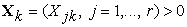to produce outputs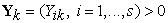. Add to the group a Perfect Object with smallest inputs and greatest outputs, and denoted as DMU0. Then, for each k = 1,…, N , we get: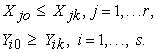(4)

When it is unambiguous, we drop the lower index k for readability. The values of i and j providing maximum values to the ratios Yi/Y0i and X0j/Xj for DMUk are labeled as i* and j*, respectively: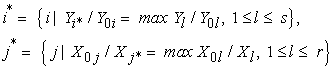(5)

The following theorem was proved in :

Theorem. Let i* and j* be unique for each DMU in a group. Then the IM CRS PO and OM CRS PO efficiency scores are equal to each other and may be found as: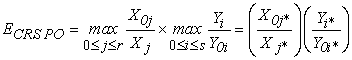(6)

where IM, OM, and CRS stand for input minimization, output maximization, and constant returns to scale, respectively.

In case of non-unique i* or j*, any of them may be used in the formula (6). For ease of notation, we will refer to ECRS PO simply as E. Figure 1 provides a geometric interpretation of the theorem for one input and one output. In this case, the IM efficiency score equals to the ratio of the distances from the y-axis to the frontier and to the DMU, while the OM efficiency, to the ratio of the distances from the x-axis to the DMU and to the frontier. The theorem follows from elementary geometric considerations.

As follows from formula (6), given the joint distribution of relative inputs and outputs, the probability distribution of the efficiency score can be found explicitly. The underlying theory and corresponding formulas can be found in . From formula (6), it follows that the efficiency scores for each DMUk k = 1,…, N are determined by the product of maximum normalized inputs and outputs. This observation allows for the introduction of stochasticity into DEA PO that is not related to the inaccuracy in the measurement of inputs or outputs. In contrast, stochastic DEA PO considers normalized actual DMUs as occurrences of identically distributed random DMUs. By doing so, it converts a group of DMUs under consideration into a statistical sample for which the probability distribution of the efficiency score can be estimated. The mathematical expectation of the group's efficiency score is referred to below as common efficiency Ec: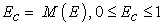(7)

where symbol M stands for the mathematical expectation. The common efficiency is the efficiency of the whole group and it serves as a benchmark for the evaluations of the partial efficiencies of the group's individual members.

Common efficiency shows how well the group is performing overall. Its use in the group-oriented approach to efficiency estimation is practical in some applications, such as a comparative study of national and regional environmental efficiency. In this case it is useful to evaluate how well a group of economies meets environmental standards, as well as efficiency variation among its members. For every particular DMUk, the difference between individual efficiency and common efficiency is referred to as partial efficiency Epk: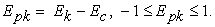(8)

As follows from formula (8), partial efficiency Epk is scaled to the interval [-1,1]. It shows how well an individual DMUk performs with regard to the group as a whole. Negative values of partial efficiency Epk mean that a corresponding DMU performs worse than the group at large and requires special attention of the regulatory body.

It should be noted that while conventional DEA efficiency scores are bound to the interval [0,1], publications  and  introduced “super efficiency” that allows it to be greater than 1. Similarly, negative values of the partial efficiency introduced in this paper extend the interval of feasible values of efficiency scores further to values below zero.

Introduction of common and partial efficiency leads to additive decomposition of the total efficiency of the DMUk as follows: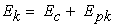(9)

where Ek, Ec , and Epk stand for the total efficiency score and its common and partial components for DMUk, respectively.

With inputs and outputs normalized to the interval [0,1] and considered as occurrences of identically distributed random objects, the Beta distribution is a convenient choice for fitting their distributions, see . The support of the Beta distribution is the interval [0,1] - same as that of the DEA PO normalized inputs and outputs. It has two parameters: α and β that control the shape of the probability density function (pdf). Depending on the values of α and β, the Beta-pdf may be increasing, decreasing, bell–shaped, U–shaped, or horizontal. A statistical computer language R provides a procedure to estimate parameters of the Beta distribution. It is available on the internet for free download .

Recall that the pdf of the Beta distribution is: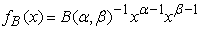(10)

where Β(α,β) is the Beta–function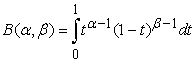(11)

The mathematical expectation and variance of the Beta distribution are as follows: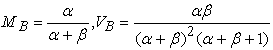(12)

A cumulative distribution function (cdf) of the maximum normalized inputs and outputs that appear in the formula (6) can be found using the formulas for the probability distribution of order statistics, see . Let ζ = <ζ1,ζ2,…,ζp> be a random vector with cdf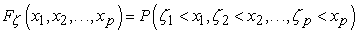(13)

and η = max(ζq,q=1,…,p). Then the cdf of the random variable η is: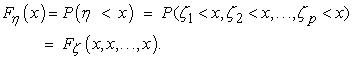(14)

A pdf of the random variable η, denoted as fη(x), can be found by partial differentiation of its cdf wth respect to x1,x2,…,xp and consequent substituting x for every xq, q = 1,…p: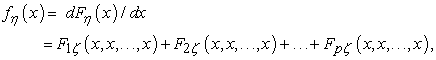(15)

where F(x,x,…,x) stands for the partial derivative of Fζ (x,x,…,x) with respect to q-th argument, q = 1,…,p.

In case of independent random variables ζ1,ζ2,…,ζp,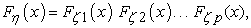(16)

so that: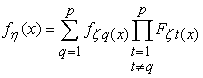(17)

where fξq(x) is a pdf of the random variable ζq. In the case of two inputs and two outputs considered in the next section, this formula becomes: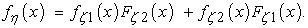(18)

For three inputs or outputs, the formula is: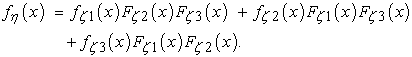(19)

It may be noted that the number of additive terms in formulas (16) through (19) grows linearly with the number of inputs or outputs, and their computation requires just minor changes in the computer program.

In addition to the pdf of the maximum value, a formula (6) also requires computation of the pdf of the product of two random variables having support [0,1]. Designating the pdf of the joint distribution of the factors as g(x,y), we get ,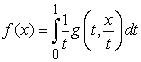(20)

that simplifies to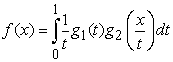(21)

for two independent random variables.

Publication  emphasizes computational problems that may arise when formulas (20) or (21) are used in practice. However, these problems may be avoided if inputs and outputs are independent. In this case, the mathematical expectation of the product of the maxima of normalized inputs and outputs equals to the product of their mathematical expectations: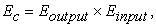(22)

where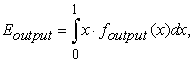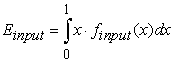(23)

where Eoutput and Einput are calculated using a formula (18) for two elements, formula (19) for three, and formula (17), for a greater number of the elements. Formula (22) holds for any number of inputs and outputs.

Formulas (17), (18), (19), (22) and (23) shed light on how SDEA PO operates, and on its ability to fix a problem related to conventional DEA revealed in the literature. As mentioned in literature - , for example - DEA may assign an efficiency score of 1 (fully efficient) to an object with a single very large output or a very small input. Similarly, DEA PO may assign a high efficiency score to an object having just one very large output and one very small input. These observations may undermine the ability of DEA to assign ranks objectively. Contrary to the deterministic versions, stochastic DEA PO, when producing a common efficiency score Ec, blends all of the indicators of all objects. By doing so, it obviates an opportunity for any single input or output to have a decisive impact.

The steps performed by the SDEA PO on the way to finding the common efficiency Ec are as follows. First, it blends inputs and outputs into two random vectors, with each component corresponding to one input or output, respectively. Next, it fits the Beta - distribution to the sample, and uses the distributions of inputs and outputs to obtain the pdf's of maximum values of relative inputs and outputs – finput and foutput – as shown by formulas (17), (18) and (19). Then the mathematical expectations Eoutput and Einput, as given by formulas (23), are produced. Finally, the common efficiency score Ec is obtained as a product of two mathematical expectations, see formula (22) - a stochastic equivalent of formula (6). As a result, all inputs and outputs, rather than the maxima only, are included in the common efficiency score Ec.

It may be noted that computationally SDEA PO is robust with regard to the numbers of inputs and outputs. Each additional input or output just adds one more additive term in the structure of formula (17) and one multiplicative term to each addend.

3. Example of Applications

This section presents an example of application of the SDEA PO to the prospective comparative analysis of environmental efficiency of major national and regional economies. While conventional DEA is widely used in environmental performance research, see , for example, SDEA PO extends the opportunities in this arena. In this section, we analyze how well a group of major national and regional economies is expected to perform from the environmental point of view, and which of them need improvement. In calculations, we use prospective data for 2030, available on the website of the Energy Information Administration of the United States . The website provides prospective data for GDP, population, total primary energy consumption, and carbon dioxide emissions. The data on the area were collected from the website of the United Nations Statistics Division .

Efficiency scores obtained by using a DEA PO or an SDEA PO are independent of the units of measurement. For convenience, we transformed the quantitative indicators into the percentage of the world total, as shown in Table 1. A select group of objects includes major national and regional economies that cover 80% to 94% of the total for each quantitative indicator. At the next step, the data in Table 1 were further transformed into the ratios presented in Table 2, columns (2) to (5), similar to that suggested in .

In general, given five quantitative indicators: Gross Domestic Product (G), Population (P), Area (A), Primary Energy Consumption (R), and Equivalent CO2 Emissions (C), there are 20 ratios available for inclusion in the DEA model, as shown in Table 3. However, most of these ratios are functionally dependent. Thus, the ratios that are symmetrical about the main diagonal of Table 3 are inverses of each other. For example, R/C = 1/(C/R). Also, any three ratios that form an L-shape in Table 3 are interconnected as well. For instance, (P/R)(A/P) = (A/R). It may be shown that with five quantitative indicators, there are only four functionally independent ratios.

In this paper, we used functionally independent ratios with clear economic interpretations referred to below as environmental ratios. Among them are Energy intensity of GDP (R/G) and Emissions intensity of energy (C/R) – the ratios typical for environmental studies. They were used as DEA inputs. For outputs, we used GDP per capita (G/P), a commonly used economic indicator, and the ratio of the Area to CO2-equivalent emissions (A/C). The last ratio is atmospheric clearness of a country or region. By choosing it, we stress the responsibility of each country or region for the atmospheric quality within its borders.

Functional independence of the selected ratios can be proven by using a functional determinant of the Jacobian matrix . To prove it, we consider these ratios as functions of the quantitative indicators C, A, R, P, and G, that is h1 = G/P, h2 = A/C, h3 = R/G, and h4 = C/R. With straightforward calculations, it can be shown that the Jacobian matrix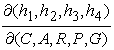has rank 4, so that the functions hi , i=1,..4, and, therefore corresponding environmental ratios, are functionally independent.

Columns (6) to (9) in Table 2 present normalized environmental ratios obtained as the ratios of i-output to maximum output, and of minimum input to j-input, respectively. They were used further as DEA PO inputs and outputs. For example, for the United States, the normalized values of inputs and outputs are as follows: output1 = 3.6723/3.6723 = 1.0000, output2 = 0.4141/5.8420 = 0.0709, input1 = 0.6643/1.0174 = 0.6529, and input2 = 0.6634/0.9278 = 0.7150. Maxima of the two normalized outputs and inputs are given in columns (10) and (11), while column (12) presents their product expressed in percent. For instance, for the U.S., max(1.0000, 0.0709) = 1.000, max(0.6529, 0.7150) = 0.7150. The product is 1.0 × 0.7150 = 0.7150 or 71.50%, which is the total efficiency score calculated by the formula (6) and shown in column (12) of Table 2 in the row corresponding to the U.S.

Table 1. Projections for 2030, % of the World Total

For analysis, we used SDEA PO to separate total efficiency into additive components: common and partial efficiency, as given by the formula (9). The common component Ec is a part of the total efficiency; it was calculated using SDEA PO and a computer program provided in the Appendix section. Methodologically, Ec corresponds to the worldwide environmental efficiency level obtained by weighing CO2-equivalent emissions against economic development, technological progress, energy consumption, area, and population. It was calculated by fitting the Beta distribution to the normalized environmental ratios and then by using formula (18) for finding the probability distribution of the corresponding maxima, and formulas (23) and (22) for calculations of the mathematical expectations and common efficiency, respectively. For the calculations, we used a program written by the author in R - language, version R 2.13.0 given in the Appendix.

While the R language procedure “fitdist” with default parameters (maximum likelihood method) gave the best results in simulations, it failed to fit to actual data. Because of that, we used the program parameters providing maximum goodness of fit estimation (MGE) using the Cramér–von Mises (CvM) distance. Table 4 presents the values of the parameters α and β for the Beta distribution. These values were used in the pdf functions g1 and g2 in formula (10). By doing so, we obtained the common efficiency score Ec = 46.43%. This value was used in column 13 of Table 2 to calculate partial efficiency scores as the difference between total and common efficiency, see formula (8).

Table 4. Beta-distribution parameters, 2030

Figure 2 shows total and partial efficiency graphically. As follows from Table 2 and Figure 2, the U.S. and Canada are expected to have high values of total efficiency (71.50% and 82.17%, respectively), and positive values of partial efficiency (25.07% and 35.74%, respectively). For the OECD countries of Europe and Australia/New Zealand region, the expected total efficiency is sufficiently lower: 56.11% and 58.57%, respectively, though partial efficiency is still positive - 9.68% and 12.14%, respectively. When looking at the BRIC countries (Brazil, Russia, India, and China) that are conventionally considered as the leading force of future economic development, it may be noted that only Brazil is expected to have positive partial efficiency of 9.05%. The other three bear negative values: Russia -4.04%, China -29.81, and India -35.61%. The Middle East is another region with a large negative partial efficiency score of -27.57%.

Obtained results may be used to develop recommendations on economic restructuring leading to better environmental performance worldwide.

4. Conclusions

The paper introduces Stochastic DEA with a Perfect Object (SDEA PO) and demonstrates its application to prospective analysis of environmental efficiency.

SDEA PO considers a group of DMUs as a sample of identically-distributed normalized DMU occurrences. An explicit formula derived for the DEA PO efficiency index allows us to obtain the probability distribution of the group’s efficiency score. The mathematical expectation of this score characterizes the efficiency of the group at large and is called common efficiency. The deviation of the observed or prospective efficiency of a particular DMU from the group level is called partial efficiency. It reflects the relative performance of a specific DMU in the group. The SDEA PO approach is applied to the analysis of environmental efficiency of major national and regional economies. It reveals how different national and regional economies perform and can aid in the development of clean air policies.

Acknowledgement

Partial support for this research was provided by a PSC-CUNY Award # 65135-00 43, jointly funded by The Professional Staff Congress and The City University of New York. Author is thankful to the anonymous reviewer for useful comments.

References

  Andersen, P., Petersen, N., “A procedure for ranking efficient units in data envelopment analysis,” Management Science, 39(10), 1261-1264, 1993.In article CrossRef  Banker, R., Stochastic data envelopment analysis. Carnegie-Mellon University, Pittsburgh, 1986.In article PubMed  Banker, R. D., Charnes, A., Cooper, W., “Some models for estimating technical and scale efficiency in Data Envelopment Analysis,” Management Science, 30(9), 1078-1092, 1984.In article CrossRef  Charnes, A., Cooper, W., "Programming with linear fractional functionals," Naval Research Logistics Quarterly, 9, 181-186, 1962.In article CrossRef  Charnes, A., Cooper, W., Rhodes, E., “Measuring the efficiency of decision-making units,” European Journal of Operational Research, 2, 429-444, 1978.In article CrossRef  Cooper, W., Seiford L., and Tone, K., Data Envelopment Analysis: A comprehensive text with models, applications, references and DEA-Solver software. (2nd Ed). New York: Springer, 2006.In article  Farrell, M. "The measurement of production efficiency,” Journal of the Royal Statistical Society, Series A, 120 (3), 253-282, 1957.In article CrossRef  Evans, M., Hastings, N., & Peacock, B., “Beta Distribution”, In: Statistical Distributions, 3rd Ed. New York: Wiley, p. 34-42, (Chapter 5), 2000.In article PubMed  Glenn, A., Evans, D., and Leemis, L., “APPL: A Probability Programming Language,” The American Statistician, 55 (2), 156-166, 2001.In article CrossRef  Gstach, D., “Another approach to data envelopment analysis in noisy environments: DEA+,” Journal of Productivity Analysis, 9, 161–176, 1998.In article CrossRef  ICON Group International, Data Envelopment Analysis: Webster's Timeline History, 1981-2007. ICON Group International, Inc., 2009.In article  Kaplan W., Advanced calculus. (4th Ed.) New York: Addison-Wesley, 1993.In article  Khodabakhshi, M., Asgharian, M., Gregoriou, G., “An input - oriented super-efficiency measure in stochastic data envelopment analysis: Evaluating chief executive officers of US public banks and thrifts. Expert Systems with Applications, 37, 2092-2097, 2010.In article CrossRef  Mereste U., “On the matrix method of analysis of economic efficiency of social production,” (“O matrichnom metode analiza ekonomicheskoi effectivnosti obshestvennogo proizvodstva”), Ekonomika i matematicheskie metody, XVIII (1), 138-49, 1982. (in Russian).In article  Sengupta, J., “Efficiency measurement in stochastic input-output systems,” International Journal of Systems Science, 13, 273-287, 1982.In article CrossRef  Spiegel, M., Schiller, J., and Srinivasan, R., Probability and Statistics.( 2nd Ed.), Schaum’s Outlines. New York: McGraw Hill, 2000.In article  Vaninsky, A., “Environmental Performance of the United States Energy Sector: A DEA Model with Non-Discretionary Factors and Perfect Object,” Publications of the World Academy of Science. Engineering and Technology, 30, 139-144, 2009.In article  Vaninsky, A., “DEA with a Perfect Object: Analytical Solutions,” Communications in Mathematics and Applications, 2(1), 1-13, 2011.In article  Vaninsky, A. "Explicit formulas for efficiency scores and weight coefficients in DEA problems with a perfect object," International Journal of Mathematical Modeling, Simulation and Applications 4(3): 217-233, 2011.In article  Zhou P, Ang B., Poh KL., “Measuring environmental performance under different environmental DEA technologies,” Energy Economics, 30, 1-14, 2008.In article CrossRef  www.eia.gov - the website of the U.S. Energy Information Administration (EIA).In article  http://www.r-project.org/ - the website concerning R -language.In article  http://unstats.un.org/unsd/default.htm - the website of the United Nations Statistics Division.In article

Appendix

This section presents a program in the R language, version R 2.13.0, for fitting parameters of the Beta distribution to statistical data for two inputs and two outputs. In case of a greater number of inputs or outputs, the functions maxoutpdf and maxinppdf need be changed appropriately, as given in the text by formulas (17) or (19). In this version of the program, the statistical data were included in the R script file. In general, they should be imported from a spreadsheet or database using R language tools. Symbol “#” stands for a comment line or a part of it.

library (fitdistrplus) #MML

#---------------------- DEA Outputs --------------------------

#GDP/Pop 2030

d1<-c(1.000000, 0.860593, 0.390419, 0.670897, 0.904363, 0.562622, 0.299780, 0.108168, 0.286214, 0.064733, 0.274090, 0.238058)

#vv1<-fitdist(d1,"beta")

vv1<-fitdist(d1,"beta", method="mge ", gof="CvM")

a1=vv1\$estimate[]

b1=vv1\$estimate[]

a1

b1

#--------------------------------------------------------------

#Area/CO2 2030

d2<-c(0.070886, 0.618005, 0.162977, 0.051930, 0.702954, 0.406162, 0.037869, 0.071951, 0.143808, 1.000000, 0.554806, 0.462710)

#vv2<-fitdist(d2,"beta")

vv2<-fitdist(d2,"beta", method="mge", gof="CvM")

a2=vv2\$estimate[]

b2=vv2\$estimate[]

a2

b2

#----------------------------------------------------------------

#---------------------- DEA Inputs --------------------------

#Energy/GDP 2030

d3<- c(0.652922, 0.367918, 0.994173, 0.836308, 0.647689, 0.371945, 0.554360, 1.000000, 0.439888, 0.894605, 0.712715, 0.831749)

#vv3<-fitdist(d3,"beta")

vv3<-fitdist(d3,"beta", method="mge", gof="CvM")

a3=vv3\$estimate[]

b3=vv3\$estimate[]

a3

b3

#--------------------------------------------------------------

#CO2/Energy 2030

d4<-c(0.714979, 0.954810, 0.705225, 0.796809, 0.625570, 0.753452, 0.523762, 0.602941, 0.658813, 0.614608, 1.000000, 0.791293)

#vv4<-fitdist(d4,"beta")

vv4<-fitdist(d4,"beta", method="mge", gof="CvM")

a4=vv4\$estimate[]

b4=vv4\$estimate[]

a4

b4

#--------------------------------------------------------------

#---------- pdf's for DEA max ratios for outputs and inputs-------------------

maxoutpdf<-function(x,aa1,bb1,aa2,bb2) {dbeta(x,aa1,bb1)*pbeta(x,aa2,bb2)+dbeta(x,aa2,bb2)*pbeta(x,aa1,bb1)}

maxinppdf<-function(x,aa3,bb3,aa4,bb4) {dbeta(x,aa3,bb3)*pbeta(x,aa4,bb4)+dbeta(x,aa4,bb4)*pbeta(x,aa3,bb3)}

maxout<-function(x) {x*maxoutpdf(x,a1,b1,a2,b2)}

maxinp<-function(x) {x*maxinppdf(x,a3,b3,a4,b4)}

#---------- Mathematical expectations of max ratios of inputs and outputs ------------

effout<-integrate(maxout,0,1)

effout\$value

effinp<-integrate(maxinp,0,1)

effinp\$value

#------------------------------------------------------------------

#------------ Common efficiency ----------------------------

effcomm=effout\$value*effinp\$value

effcomm